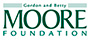Home | Activities and links | Walking Polygons

# Walking Polygons

Investigate the interior angles of polygons with your feet.

## Background

In this activity, you’ll walk the sides and interior angles of various polygons drawn on the playground. As you do so, you will rotate. Practice rotating clockwise 180° and 360° before doing this activity.

## Materials

• playground or other paved surface
• chalk
• two or more people

## What’s Going On?

There’s a pattern to the sum of the interior angles of any polygon. In each polygon, you rotated through some multiple of 180°. The sum of the interior angles of a triangle is 180°, the sum of the interior angles of a quadrilateral is 360°, and the sum of the interior angles of a pentagon is 540°. Draw a table on the pavement to record your data. Look for a pattern between the number of sides of the polygon and the sum of its interior angles. There’s a formula that describes this pattern: For any polygon with n number of sides, the sum of the interior angles will be 180° (n – 2).

Try This
1. Draw a large chalk triangle on the pavement. Make the lengths of the sides between 1 and 2 meters long.

2. Choose one person to be the Walker. The others will be Observers who stand outside the triangle and watch the motion of the Walker.

Note: Steps 3 through 6 are directions for the Walker.

3. Notice the walking/pivoting pattern

When you are moving forward, you pivot on your toes at the vertex, ready to walk backward along the next side. When you are moving backward, you pivot on your heels at the vertex, ready to walk forward along the next side.

4. Stand inside the triangle with your right foot along the midpoint of one side. Ask an Observer to mark the location of your feet with chalk, as this will be both the starting and ending location.

5. Walk until you reach the vertex in front of you—the point where two of the lines come together—so that your toes go into the corner. To “walk” the interior angle, pivot clockwise on your toes just enough so that your heels point toward the next vertex and your left foot is lined up with the second side. (See illustrations.)6. Walk backward along this second side until your heels reach the vertex. Pivot clockwise on your heels so that your toes point toward the third vertex and your right foot is lined up with the third side.

7. Walk along the third side, pivot clockwise in the last vertex, and then finish by walking backward to the starting/ending chalk line.

8. Everyone should take note of the direction the Walker is facing and then confer about the Walker’s total rotation, remembering the 180° and 360° rotations that you practiced. You should notice that the number of degrees the Walker rotated is the sum of the interior angles of a triangle, which is 180°. One person should write “180°” inside the triangle.

9. Now draw a large quadrilateral such as a square or rectangle on the pavement.

10. Change roles, and have the new Walker walk the sides and interior angles of the quadrilateral.

11. After the Walker has returned to the starting point, discuss the total rotation for the quadrilateral. Notice that the number of degrees the Walker rotated is the sum of the interior angles of a quadrilateral, which is 360°. Write “360°” inside the quadrilateral.

12. Try walking the sides and interior angles of a pentagon and a hexagon. Do you see a pattern?

13. Choose a vertex in each of the polygons you have drawn on the playground, excluding the triangle. From that vertex, draw lines to the other vertices, except the two that are already connected. Doing this will divide each polygon into some number of triangles. Can this help you figure out the sum of the interior angles of the pentagon and the hexagon?

This activity idea is from Karl Schaffer, math professor at De Anza College in Cupertino, CA, and one of the founders of MathDance: www.mathdance.org.

Geometry Playground is made possible by the National Science Foundation and the Gordon and Betty Moore Foundation.

Any opinions, findings, conclusions, or recommendations expressed in this material are those of the author(s) and do not necessarily reflect the views of the National Science Foundation.Credits

© 2010 Exploratorium | The museum of science, art and human perception